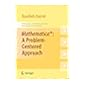Normal view

# Mathematica®: A Problem-Centered Approach [electronic resource] / by Roozbeh Hazrat.

Material type:TextPublisher: Cham : Springer International Publishing : Imprint: Springer, 2015Edition: 2nd ed. 2015Description: XIX, 318 p. 164 illus., 25 illus. in color. online resourceContent type: text Media type: computer Carrier type: online resourceISBN: 9783319275857Additional physical formats: Printed edition:: No titleDDC classification: 005.131 LOC classification: QA76.9.M35Online resources: Click here to access online
Contents:
Introduction -- Basics -- Defining functions -- Lists -- Changing heads! -- A bit of logic and set theory -- Sums and products -- Loops and repetitions -- Substitutions, Mathematica rules -- Pattern matching -- Functions with multiple definitions -- Recursive functions -- Linear algebra -- Graphics -- Calculus and equations -- Worked out projects -- Projects -- Solutions to the Exercises -- Further reading -- Bibliography -- Index.
Summary: This textbook introduces the vast array of features and powerful mathematical functions of Mathematica using a multitude of clearly presented examples and worked-out problems. Each section starts with a description of a new topic and some basic examples. The author then demonstrates the use of new commands through three categories of problems - the first category highlights those essential parts of the text that demonstrate the use of new commands in Mathematica whilst solving each problem presented; - the second comprises problems that further demonstrate the use of commands previously introduced to tackle different situations; and - the third presents more challenging problems for further study. The intention is to enable the reader to learn from the codes, thus avoiding long and exhausting explanations. While based on a computer algebra course taught to undergraduate students of mathematics, science, engineering and finance, the book also includes chapters on calculus and solving equations, and graphics, thus covering all the basic topics in Mathematica. With its strong focus upon programming and problem solving, and an emphasis on using numerical problems that do not need any particular background in mathematics, this book is also ideal for self-study and as an introduction to researchers who wish to use Mathematica as a computational tool. This new edition has been extensively revised and updated, and includes new chapters with problems and worked examples.
Tags from this library: No tags from this library for this title.Average rating: 0.0 (0 votes)
Item type Current location Collection Call number Status Date due Barcode Item holdseBook e-Library

Electronic Book@IST

EBook Available
Total holds: 0

Introduction -- Basics -- Defining functions -- Lists -- Changing heads! -- A bit of logic and set theory -- Sums and products -- Loops and repetitions -- Substitutions, Mathematica rules -- Pattern matching -- Functions with multiple definitions -- Recursive functions -- Linear algebra -- Graphics -- Calculus and equations -- Worked out projects -- Projects -- Solutions to the Exercises -- Further reading -- Bibliography -- Index.

This textbook introduces the vast array of features and powerful mathematical functions of Mathematica using a multitude of clearly presented examples and worked-out problems. Each section starts with a description of a new topic and some basic examples. The author then demonstrates the use of new commands through three categories of problems - the first category highlights those essential parts of the text that demonstrate the use of new commands in Mathematica whilst solving each problem presented; - the second comprises problems that further demonstrate the use of commands previously introduced to tackle different situations; and - the third presents more challenging problems for further study. The intention is to enable the reader to learn from the codes, thus avoiding long and exhausting explanations. While based on a computer algebra course taught to undergraduate students of mathematics, science, engineering and finance, the book also includes chapters on calculus and solving equations, and graphics, thus covering all the basic topics in Mathematica. With its strong focus upon programming and problem solving, and an emphasis on using numerical problems that do not need any particular background in mathematics, this book is also ideal for self-study and as an introduction to researchers who wish to use Mathematica as a computational tool. This new edition has been extensively revised and updated, and includes new chapters with problems and worked examples.

There are no comments for this item.Mathematica®: A Problem-Centered Approach (Springer Undergraduate Mathematics Series). by Hazrat, Roozbeh. ©2010